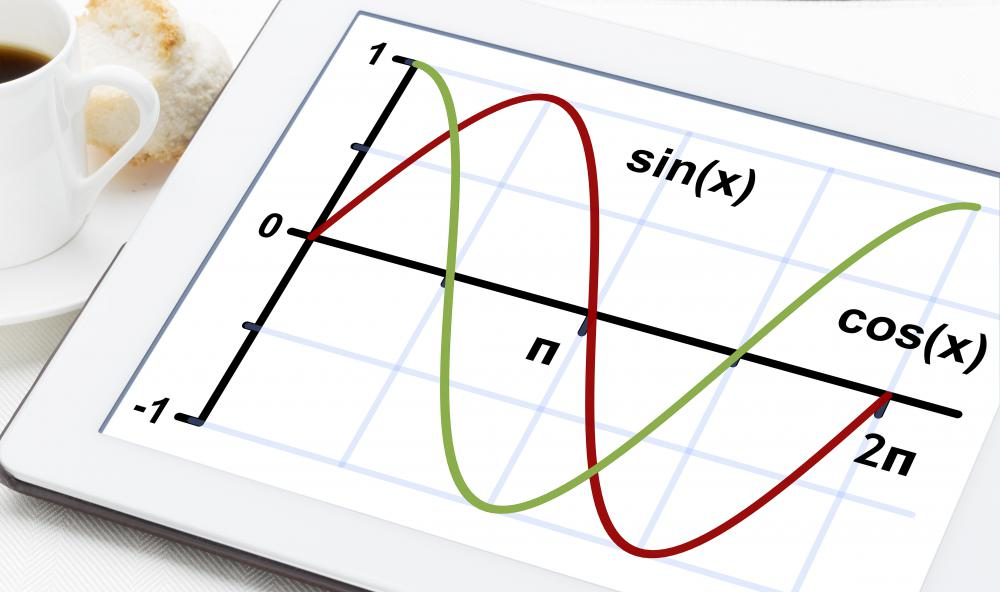# What is Trigonometry?

C. Martin

Trigonometry is a specialist branch of geometry that deals with the study of triangles. It is sometimes informally referred to as "trig." In trigonometry, mathematicians study the relationships between the sides and angles of triangles. Right triangles, which are triangles with one angle of 90 degrees, are a key area of study in this area of mathematics.In modern mathematics, there are six main trigonometric functions, also called trigonometric formulas: sine, tangent, secant, cosine, cotangent, and cosecant.

The word trigonometry is commonly believed to originate from the Greek words trigonon and metron which mean "triangle" and "measure" respectively. This is a very old science that may have been used in a basic form in ancient Egypt. It was the Greeks that formalized the first trigonometric functions, starting with Hipparchus of Bithynia in around 150 B.C.Surveyors must be able to perform complex calculations, including trigonometry and calculus, to correctly analyze a land form.

Trigonometric functions describe the relationships between the angles and sides of a triangle. In modern mathematics, there are six main trigonometric functions, also called trigonometric formulas: sine, tangent, secant, cosine, cotangent, and cosecant. These functions describe the ratios of the sides of right triangles.

Trigonometric identities are algebraic equations that are important elements of the study of triangles. Trigonometric identities include Pythagorean identities, reduction formulas, and cofunction identities. Often, a trigonometry calculator is used to solve trig problems.

Trigonometry courses may cover study topics such as how to use the trigonometric functions to solve right triangles, and the Pythagorean Theorem. In addition, non-right angled triangles can be solved using the sine and cosine trigonometric functions. More advanced educational courses may include the study of complex numbers, polar coordinates, De Moivre's Theorem, and Euler's Formula.

Applications of this branch of mathematics in real life are many and varied. Engineers in many industrial fields use trig in the course of their work. Other professionals that may use trigonometry include surveyors, astronomers, architects, and pilots.

A common real life problem that may be solved using the rules of trigonometry is to work out the measurements of things or spaces that would be difficult to measure directly in a traditional way. For example, trigonometric functions can be used to work out the heights of mountains, the quantity of water in a lake, or the square footage of a piece of land that is an unusual shape. Trigonometry can even be used to help astronomers measure time accurately.

## You might also Like